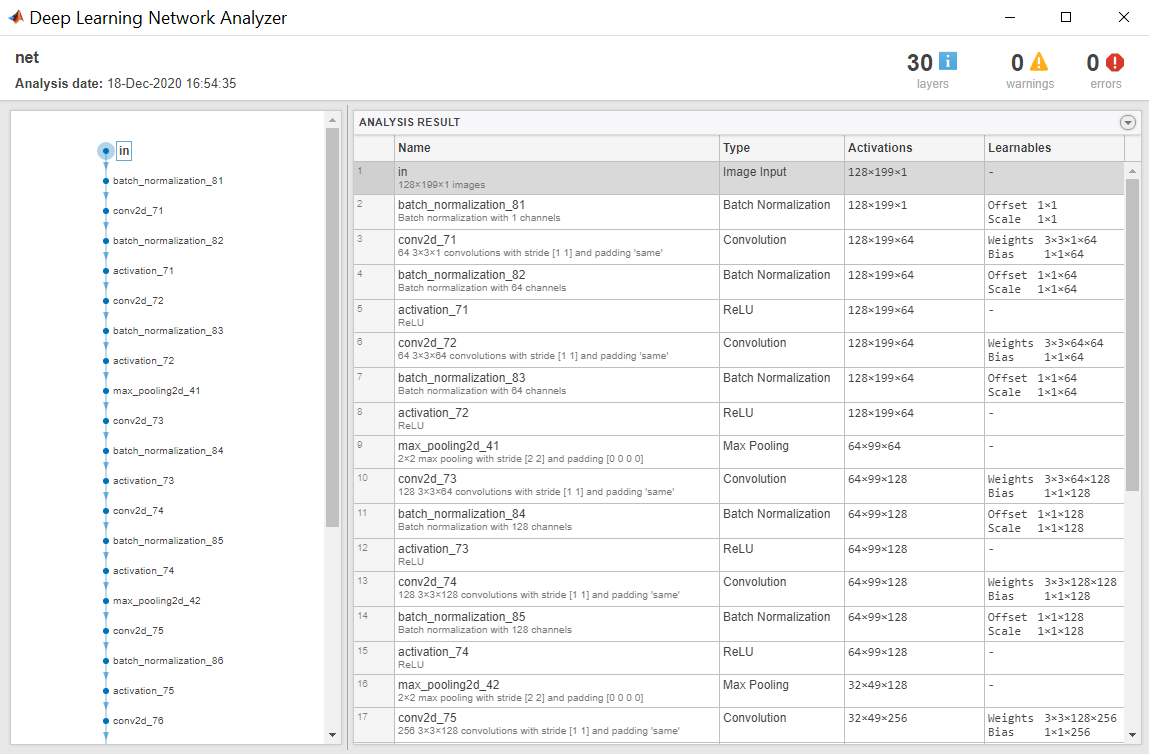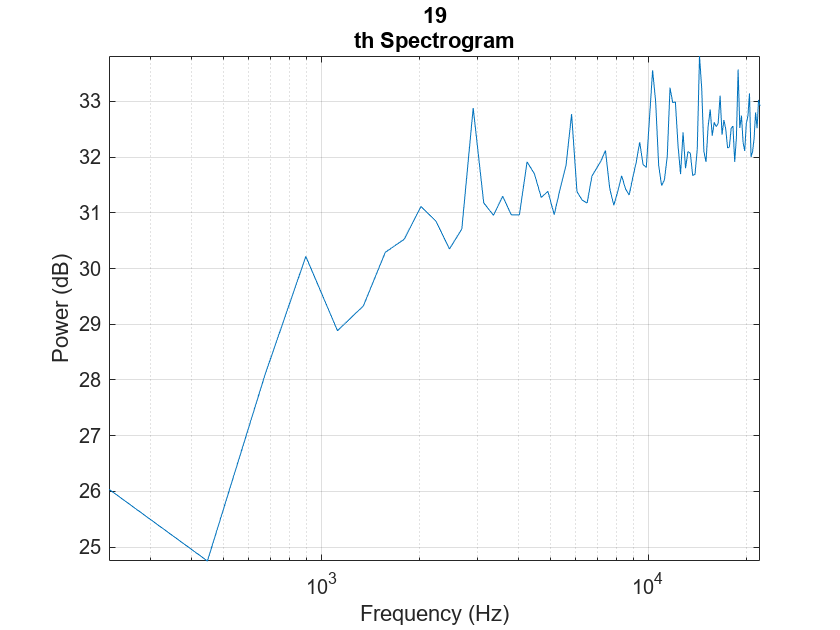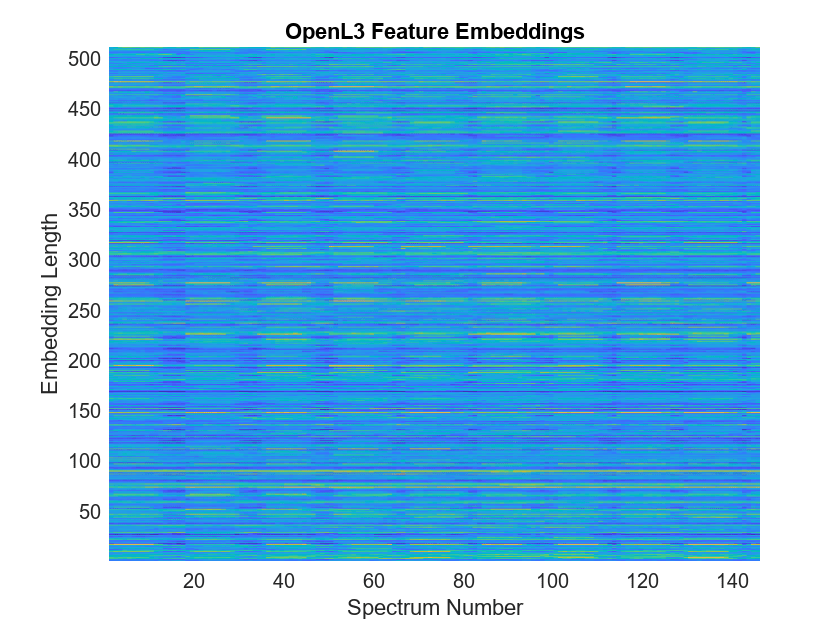# openl3

OpenL3 neural network

## Syntax

``net = openl3``
``net = openl3(Name,Value)``

## Description

example

````net = openl3` returns a pretrained OpenL3 model.This function requires both Audio Toolbox™ and Deep Learning Toolbox™.```
````net = openl3(Name,Value)` specifies options using one or more Name, Value arguments. For example, ```net = openl3('EmbeddingLength',6144)``` specifies the output embedding length as 6144.```

## Examples

collapse all

Download and unzip the Audio Toolbox™ model for OpenL3.

Type `openl3` at the Command Window. If the Audio Toolbox model for OpenL3 is not installed, the function provides a link to the location of the network weights. To download the model, click the link. Unzip the file to a location on the MATLAB path.

Alternatively, execute these commands to download and unzip the OpenL3 model to your temporary directory.

```downloadFolder = fullfile(tempdir,'OpenL3Download'); loc = websave(downloadFolder,'https://ssd.mathworks.com/supportfiles/audio/openl3.zip'); OpenL3Location = tempdir; unzip(loc,OpenL3Location) addpath(fullfile(OpenL3Location,'openl3'))```

Check that the installation is successful by typing `openl3` at the Command Window. If the network is installed, then the function returns a `DAGNetwork` (Deep Learning Toolbox) object.

`openl3`
```ans = DAGNetwork with properties: Layers: [30×1 nnet.cnn.layer.Layer] Connections: [29×2 table] InputNames: {'in'} OutputNames: {'out'} ```

Load a pretrained OpenL3 convolutional neural network and examine the layers and classes.

Use `openl3` to load the pretrained OpenL3 network. The output `net` is a `DAGNetwork` (Deep Learning Toolbox) object.

`net = openl3`
```net = DAGNetwork with properties: Layers: [30×1 nnet.cnn.layer.Layer] Connections: [29×2 table] InputNames: {'in'} OutputNames: {'out'} ```

View the network architecture using the `Layers` property. The network has 30 layers. There are 16 layers with learnable weights, of which eight are batch normalization layers and eight are convolutional layers.

`net.Layers`
```ans = 30×1 Layer array with layers: 1 'in' Image Input 128×199×1 images 2 'batch_normalization_81' Batch Normalization Batch normalization with 1 channels 3 'conv2d_71' Convolution 64 3×3×1 convolutions with stride [1 1] and padding 'same' 4 'batch_normalization_82' Batch Normalization Batch normalization with 64 channels 5 'activation_71' ReLU ReLU 6 'conv2d_72' Convolution 64 3×3×64 convolutions with stride [1 1] and padding 'same' 7 'batch_normalization_83' Batch Normalization Batch normalization with 64 channels 8 'activation_72' ReLU ReLU 9 'max_pooling2d_41' Max Pooling 2×2 max pooling with stride [2 2] and padding [0 0 0 0] 10 'conv2d_73' Convolution 128 3×3×64 convolutions with stride [1 1] and padding 'same' 11 'batch_normalization_84' Batch Normalization Batch normalization with 128 channels 12 'activation_73' ReLU ReLU 13 'conv2d_74' Convolution 128 3×3×128 convolutions with stride [1 1] and padding 'same' 14 'batch_normalization_85' Batch Normalization Batch normalization with 128 channels 15 'activation_74' ReLU ReLU 16 'max_pooling2d_42' Max Pooling 2×2 max pooling with stride [2 2] and padding [0 0 0 0] 17 'conv2d_75' Convolution 256 3×3×128 convolutions with stride [1 1] and padding 'same' 18 'batch_normalization_86' Batch Normalization Batch normalization with 256 channels 19 'activation_75' ReLU ReLU 20 'conv2d_76' Convolution 256 3×3×256 convolutions with stride [1 1] and padding 'same' 21 'batch_normalization_87' Batch Normalization Batch normalization with 256 channels 22 'activation_76' ReLU ReLU 23 'max_pooling2d_43' Max Pooling 2×2 max pooling with stride [2 2] and padding [0 0 0 0] 24 'conv2d_77' Convolution 512 3×3×256 convolutions with stride [1 1] and padding 'same' 25 'batch_normalization_88' Batch Normalization Batch normalization with 512 channels 26 'activation_77' ReLU ReLU 27 'audio_embedding_layer' Convolution 512 3×3×512 convolutions with stride [1 1] and padding 'same' 28 'max_pooling2d_44' Max Pooling 16×24 max pooling with stride [16 24] and padding 'same' 29 'flatten' Keras Flatten Flatten activations into 1-D assuming C-style (row-major) order 30 'out' Regression Output mean-squared-error ```

Use `analyzeNetwork` (Deep Learning Toolbox) to visually explore the network.

`analyzeNetwork(net)`Use `openl3Preprocess` to extract embeddings from an audio signal.

Read in an audio signal.

`[audioIn,fs] = audioread('Counting-16-44p1-mono-15secs.wav');`

To extract spectrograms from the audio, call the `openl3Preprocess` function with the audio and sample rate. Use 50% overlap and set the spectrum type to linear. The `openl3Preprocess` function returns an array of 30 spectrograms produced using an FFT length of 512.

```features = openl3Preprocess(audioIn,fs,'OverlapPercentage',50,'SpectrumType','linear'); [posFFTbinsOvLap50,numHopsOvLap50,~,numSpectOvLap50] = size(features)```
```posFFTbinsOvLap50 = 257 ```
```numHopsOvLap50 = 197 ```
```numSpectOvLap50 = 30 ```

Call `openl3Preprocess` again, this time using the default overlap of 90%. The `openl3Preprocess` function now returns an array of 146 spectrograms.

```features = openl3Preprocess(audioIn,fs,'SpectrumType','linear'); [posFFTbinsOvLap90,numHopsOvLap90,~,numSpectOvLap90] = size(features)```
```posFFTbinsOvLap90 = 257 ```
```numHopsOvLap90 = 197 ```
```numSpectOvLap90 = 146 ```

Visualize one of the spectrograms at random.

```randSpect = randi(numSpectOvLap90); viewRandSpect = features(:,:,:,randSpect); N = size(viewRandSpect,2); binsToHz = (0:N-1)*fs/N; nyquistBin = round(N/2); semilogx(binsToHz(1:nyquistBin),mag2db(abs(viewRandSpect(1:nyquistBin)))) xlabel('Frequency (Hz)') ylabel('Power (dB)'); title([num2str(randSpect),'th Spectrogram']) axis tight grid on```Create an OpenL3 network (this requires Deep Learning Toolbox) using the same `'SpectrumType'`.

`net = openl3('SpectrumType','linear');`

Extract and visualize the audio embeddings.

```embeddings = predict(net,features); surf(embeddings,'EdgeColor','none') view([90,-90]) axis([1 numSpectOvLap90 1 numSpectOvLap90]) xlabel('Embedding Length') ylabel('Spectrum Number') title('OpenL3 Feature Embeddings') axis tight```## Input Arguments

collapse all

### Name-Value Arguments

Specify optional pairs of arguments as `Name1=Value1,...,NameN=ValueN`, where `Name` is the argument name and `Value` is the corresponding value. Name-value arguments must appear after other arguments, but the order of the pairs does not matter.

Before R2021a, use commas to separate each name and value, and enclose `Name` in quotes.

Example: `openl3('EmbeddingLength',6144)`

Spectrum type generated from audio and used as input to the neural network, specified as `'mel128'`, `'mel256'`, or `'linear'`.

When using `'SpectrumType'` and:

• `'mel128'` –– The network accepts mel spectrograms with 128 mel bands as input. The input dimensions to the network are `128`-by-`199`-by-`1`-by-K, where `128` is the number of mel bands and `199` is the number of time hops.

• `'mel256'` –– The network accepts mel spectrograms with 256 mel bands as input. The input dimensions to the network are `256`-by-`199`-by-`1`-by-K, where `256` is the number of mel bands and `199` is the number of time hops.

• `'linear'` –– The network accepts positive one-sided spectrograms with an FFT length of 257. The input dimensions to the network are `257`-by-`197`-by-`1`-by-K, where `257` is the positive one-sided FFT length and `197` is the number of time hops.

K represents the number of spectrograms. When preprocessing your data with `openl3Preprocess`, you must use the same `'SpectrumType'`.

Data Types: `char` | `string`

Length of the output audio embedding, specified as `512` or `6144`.

Data Types: `single` | `double`

Audio content type the neural network is trained on, specified as `'env'` or `'music'`.

Set `ContentType` to:

• `'env'` when you want to use a model trained on environmental data.

• `'music'` when you want to use a model trained on musical data.

Data Types: `char` | `string`

## Output Arguments

collapse all

Pretrained OpenL3 neural network, returned as a `DAGNetwork` (Deep Learning Toolbox) object.

 Cramer, Jason, et al. "Look, Listen, and Learn More: Design Choices for Deep Audio Embeddings." In ICASSP 2019 IEEE International Conference on Acoustics, Speech and Signal Processing (ICASSP), IEEE, 2019, pp. 3852-56. DOI.org (Crossref), doi:/10.1109/ICASSP.2019.8682475.